•字体
•Python
• ## Pytorch详解NLLLoss和CrossEntropyLoss

万次阅读 多人点赞 2018-12-24 10:46:12
CrossEntropyLoss CrossEntropyLoss就是把以上Softmax–Log–NLLLoss合并成一步，我们用刚刚随机出来的input直接验证一下结果是不是1.0128： 真的是1.0128哈哈哈哈！我也太厉害了吧！ 如果你也觉得我很厉害，请奖励...
pytorch的官方文档写的也太简陋了吧…害我看了这么久…
NLLLoss
在图片单标签分类时，输入m张图片，输出一个m*N的Tensor，其中N是分类个数。比如输入3张图片，分三类，最后的输出是一个3*3的Tensor，举个例子：第123行分别是第123张图片的结果，假设第123列分别是猫、狗和猪的分类得分。 可以看出模型认为第123张都更可能是猫。 然后对每一行使用Softmax，这样可以得到每张图片的概率分布。这里dim的意思是计算Softmax的维度，这里设置dim=1，可以看到每一行的加和为1。比如第一行0.6600+0.0570+0.2830=1。如果设置dim=0，就是一列的和为1。比如第一列0.2212+0.3050+0.4738=1。 我们这里一张图片是一行，所以dim应该设置为1。 然后对Softmax的结果取自然对数：Softmax后的数值都在0~1之间，所以ln之后值域是负无穷到0。 NLLLoss的结果就是把上面的输出与Label对应的那个值拿出来，再去掉负号，再求均值。 假设我们现在Target是[0,2,1]（第一张图片是猫，第二张是猪，第三张是狗）。第一行取第0个元素，第二行取第2个，第三行取第1个，去掉负号，结果是：[0.4155,1.0945,1.5285]。再求个均值，结果是：下面使用NLLLoss函数验证一下：嘻嘻，果然是1.0128！
CrossEntropyLoss
CrossEntropyLoss就是把以上Softmax–Log–NLLLoss合并成一步，我们用刚刚随机出来的input直接验证一下结果是不是1.0128：真的是1.0128哈哈哈哈！我也太厉害了吧！
如果你也觉得我很厉害，请打赏以鼓励我做的更好，非常感谢！展开全文深度学习 Pytorch Loss 损失函数
• Information Entropy Information Entropy measures the information missing before reception, saying the level of uncertainty of a random variable XXX. Information entropy definition: H(X)=−∑i=1np...
Information Entropy
Information Entropy measures the information missing before reception, saying the level of uncertainty of a random variable

X

X

$X$.
Information entropy definition:
H(X)=−∑i=1np(xi)logp(xi)$H\left(X\right)=-\sum _{i=1}^{n}p\left({x}_{i}\right)\mathrm{log}p\left({x}_{i}\right)$H(X)=-\sum_{i=1}^{n}p(x_i)\operatorname{log}p(x_i)  where,

X

X

$X$ is a random variable, p(xi)$p\left({x}_{i}\right)$$p(x_i)$ is the probability of

X=xi

X

=

x

i

$X=x_i$. When

log

l

o

g

$log$ is

log2

l

o

g

2

$log_2$ the unit of

H(X)

H

(

X

)

$H(X)$ is bit. When

log

l

o

g

$log$ is

log10

l

o

g

10

$log_{10}$ the unit of

H(X)

H

(

X

)

$H(X)$ is dit.
Example
English character

X

X

$X$ is a random variable. It could be one character of a,b,c...x,y,z$a,b,c...x,y,z$$a, b, c ... x, y, z$. The information entropy of

X

X

$X$:
H(X)=−∑i=126126∗log2126=4.7$\begin{array}{rl}H\left(X\right)& =-\sum _{i=1}^{26}\frac{1}{26}\ast \mathrm{l}\mathrm{o}{\mathrm{g}}_{2}\frac{1}{26}\\ & =4.7\end{array}$ \begin{aligned}
H(X)&=-\sum_{i=1}^{26}\frac{1}{26}*\operatorname{log_2}\frac{1}{26}\\
&=4.7\\
\end{aligned}  This means the information entropy of a English character is 4.7 bit, meaning 5 binary numbers are able to encode a English character.
ASCII code

X

X

$X$ is a random variable. It could be one ASCII code. The total number of ASCII code is 128. The information entropy of X$X$$X$:

H(X)=−∑i=11281128∗log21128=7

H

(

X

)

=

−

∑

i

=

1

128

1

128

∗

l

o

g

2

1

128

=

7

\begin{aligned}
H(X)&=-\sum_{i=1}^{128}\frac{1}{128}*log_2\frac{1}{128}\\
&=7\\
\end{aligned}
This means the information entropy of a English character is 7 bit, meaning 7 binary numbers are able to encode an ASCII code. We use a Byte, which is 8 bits, to stand for a ASCII code. The extra one bit is used for checking.

================================================
Cross Entropy in Machine Learning
In information theory, the cross entropy between two probability distributions

p

p

$p$ and q$q$$q$ over the same underlying set of events measures the average number of bits needed to identify an event drawn from the set, if a coding scheme is used that is optimized for an “unnatural” probability distribution

q

q

$q$, rather than the “true” distribution p$p$$p$.  Cross entropy definition:

S(p,q)=−∑xp(x)logq(x)

S

(

p

,

q

)

=

−

∑

x

p

(

x

)

log

⁡

q

(

x

)

S(p, q)=-\sum_{x}p(x)\operatorname{log}q(x)
where,

x

x

$x$ is each certain value of the set.p$p$$p$ is the target distribution,

q

q

$q$ is the temporary, unreal or unnatural distribution.

The more similar p$p$$p$ and

q

q

$q$, the smaller S(p,q)$S\left(p,q\right)$$S(p, q)$. So

S(.)

S

(

.

)

$S(.)$ could be used as training target. There is an application, named
tf.nn.softmax_cross_entropy_with_logits_v2(), in tensorflow for this.

Example
The training instance one-hot label is

y_target=[0,1,0,0,0]

y

_

t

a

r

g

e

t

=

[

0

,

1

,

0

,

0

,

0

]

$y\_target=[0, 1, 0, 0, 0]$. The one-hot label calculated by you algorithm

y_tmp=[0.1,0.1,0.2,0.1,0.5]

y

_

t

m

p

=

[

0.1

,

0.1

,

0.2

,

0.1

,

0.5

]

$y\_tmp=[0.1, 0.1, 0.2, 0.1, 0.5]$.
You want to make

y_tmp

y

_

t

m

p

$y\_tmp$ approximating

y_target

y

_

t

a

r

g

e

t

$y\_target$. In other word, you goal is to make

y_tmp

y

_

t

m

p

[

0

]

$y\_tmp$ smaller, to make

y_tmp

y

_

t

m

p

[

1

]

$y\_tmp$ greater, to make

y_tmp

y

_

t

m

p

[

2

]

$y\_tmp$ smaller … It’s a complex task. so much to be consider.

S(y_target,y_tmp)

S

(

y

_

t

a

r

g

e

t

,

y

_

t

m

p

)

$S(y\_target, y\_tmp)$ be smaller? One shot all done. Better.
Ref
Cross entropy - Wikipedia  https://en.wikipedia.org/wiki/Cross_entropy
A Friendly Introduction to Cross-Entropy Loss  https://rdipietro.github.io/friendly-intro-to-cross-entropy-loss/#entropy
Entropy - Wikipedia  https://en.wikipedia.org/wiki/Entropy
展开全文•熵值法
• a maximum entropy approach to natrual language processing L.BERGET 大神关于最大熵模型的著作。
• Overcoming Weak Expectations via the Renyi Entropy and the Expanded Computational Entropy研究论文
• 我们知道卷积神经网络（CNN）在图像领域的应用已经非常广泛了，一般一个CNN网络主要包含卷积层，池化层...你对softmax，softmax loss，cross entropy了解吗？相信很多人不一定清楚。虽然网上的资料很多，但是...
我们知道卷积神经网络（CNN）在图像领域的应用已经非常广泛了，一般一个CNN网络主要包含卷积层，池化层（pooling），全连接层，损失层等。虽然现在已经开源了很多深度学习框架（比如MxNet，Caffe等），训练一个模型变得非常简单，但是你对这些层具体是怎么实现的了解吗？你对softmax，softmax loss，cross entropy了解吗？相信很多人不一定清楚。虽然网上的资料很多，但是质量参差不齐，常常看得眼花缭乱。为了让大家少走弯路，特地整理了下这些知识点的来龙去脉，希望不仅帮助自己巩固知识，也能帮到他人理解这些内容。
这一篇主要介绍全连接层和损失层的内容，算是网络里面比较基础的一块内容。先理清下从全连接层到损失层之间的计算。来看下面这张图，来自参考资料1（自己实在懒得画图了）。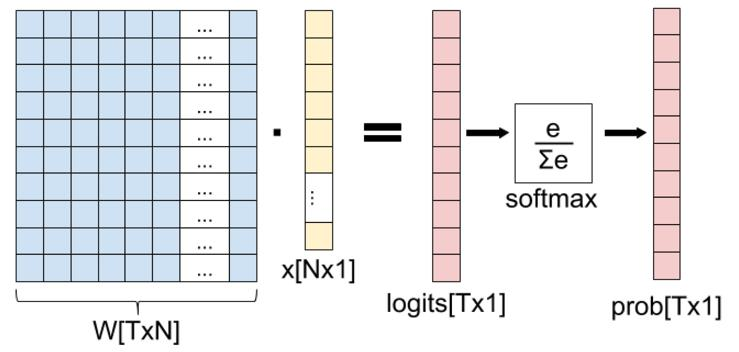这张图的等号左边部分就是全连接层做的事，W是全连接层的参数，我们也称为权值，X是全连接层的输入，也就是特征。从图上可以看出特征X是N*1的向量，这是怎么得到的呢？这个特征就是由全连接层前面多个卷积层和池化层处理后得到的，假设全连接层前面连接的是一个卷积层，这个卷积层的输出是100个特征（也就是我们常说的feature map的channel为100），每个特征的大小是4*4，那么在将这些特征输入给全连接层之前会将这些特征flat成N*1的向量（这个时候N就是100*4*4=1600）。解释完X，再来看W，W是全连接层的参数，是个T*N的矩阵，这个N和X的N对应，T表示类别数，比如你是7分类，那么T就是7。我们所说的训练一个网络，对于全连接层而言就是寻找最合适的W矩阵。因此全连接层就是执行WX得到一个T*1的向量（也就是图中的logits[T*1]），这个向量里面的每个数都没有大小限制的，也就是从负无穷大到正无穷大。然后如果你是多分类问题，一般会在全连接层后面接一个softmax层，这个softmax的输入是T*1的向量，输出也是T*1的向量（也就是图中的prob[T*1]，这个向量的每个值表示这个样本属于每个类的概率），只不过输出的向量的每个值的大小范围为0到1。
现在你知道softmax的输出向量是什么意思了，就是概率，该样本属于各个类的概率！
那么softmax执行了什么操作可以得到0到1的概率呢？先来看看softmax的公式（以前自己看这些内容时候对公式也很反感，不过静下心来看就好了）：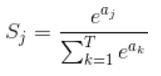公式非常简单，前面说过softmax的输入是WX，假设模型的输入样本是I，讨论一个3分类问题（类别用1，2，3表示），样本I的真实类别是2，那么这个样本I经过网络所有层到达softmax层之前就得到了WX，也就是说WX是一个3*1的向量，那么上面公式中的aj就表示这个3*1的向量中的第j个值（最后会得到S1，S2，S3）；而分母中的ak则表示3*1的向量中的3个值，所以会有个求和符号（这里求和是k从1到T，T和上面图中的T是对应相等的，也就是类别数的意思，j的范围也是1到T）。因为e^x恒大于0，所以分子永远是正数，分母又是多个正数的和，所以分母也肯定是正数，因此Sj是正数，而且范围是(0,1)。如果现在不是在训练模型，而是在测试模型，那么当一个样本经过softmax层并输出一个T*1的向量时，就会取这个向量中值最大的那个数的index作为这个样本的预测标签。
因此我们训练全连接层的W的目标就是使得其输出的WX在经过softmax层计算后其对应于真实标签的预测概率要最高。
举个例子：假设你的WX=[1,2,3]，那么经过softmax层后就会得到[0.09,0.24,0.67]，这三个数字表示这个样本属于第1,2,3类的概率分别是0.09,0.24,0.67。
———————————–华丽的分割线——————————————
弄懂了softmax，就要来说说softmax loss了。  那softmax loss是什么意思呢?如下：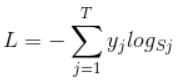首先L是损失。Sj是softmax的输出向量S的第j个值，前面已经介绍过了，表示的是这个样本属于第j个类别的概率。yj前面有个求和符号，j的范围也是1到类别数T，因此y是一个1*T的向量，里面的T个值，而且只有1个值是1，其他T-1个值都是0。那么哪个位置的值是1呢？答案是真实标签对应的位置的那个值是1，其他都是0。所以这个公式其实有一个更简单的形式：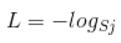当然此时要限定j是指向当前样本的真实标签。
来举个例子吧。假设一个5分类问题，然后一个样本I的标签y=[0,0,0,1,0]，也就是说样本I的真实标签是4，假设模型预测的结果概率（softmax的输出）p=[0.1,0.15,0.05,0.6,0.1]，可以看出这个预测是对的，那么对应的损失L=-log(0.6)，也就是当这个样本经过这样的网络参数产生这样的预测p时，它的损失是-log(0.6)。那么假设p=[0.15,0.2,0.4,0.1,0.15]，这个预测结果就很离谱了，因为真实标签是4，而你觉得这个样本是4的概率只有0.1（远不如其他概率高，如果是在测试阶段，那么模型就会预测该样本属于类别3），对应损失L=-log(0.1)。那么假设p=[0.05,0.15,0.4,0.3,0.1]，这个预测结果虽然也错了，但是没有前面那个那么离谱，对应的损失L=-log(0.3)。我们知道log函数在输入小于1的时候是个负数，而且log函数是递增函数，所以-log(0.6) < -log(0.3) < -log(0.1)。简单讲就是你预测错比预测对的损失要大，预测错得离谱比预测错得轻微的损失要大。
———————————–华丽的分割线———————————–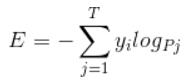是不是觉得和softmax loss的公式很像。当cross entropy的输入P是softmax的输出时，cross entropy等于softmax loss。Pj是输入的概率向量P的第j个值，所以如果你的概率是通过softmax公式得到的，那么cross entropy就是softmax loss。这是我自己的理解，如果有误请纠正。
下一篇将介绍 卷积神经网络系列之softmax loss对输入的求导推导
参考资料1：http://eli.thegreenplace.net/2016/the-softmax-function-and-its-derivative/
展开全文深度学习
• In this course, there is an important chapter about information entropy. Entropy is the average amount of information contained in each message received. Here, a message stands for an event, or a ...character less random string 函数
• Regularized discriminant entropy analysis研究论文
• here is an ongoing research effort devoted to characterize the signal regularity metrics approximate entropy (ApEn) and sample entropy (SampEn) in order to better interpret their results in the ...
• HIGHLIGHTS: Twelve entropy indices were systematically compared in monitoring depth of anesthesia and detecting burst suppression. Renyi permutation entropy performed best in tracking EEG changes ...
•JavaScript
• 在使用Pytorch时经常碰见这些函数cross_entropy，CrossEntropyLoss, log_softmax, softmax。看得我头大，所以整理本文以备日后查阅。 首先要知道上面提到的这些函数一部分是来自于torch.nn,而另一部分则来自于...

在使用Pytorch时经常碰见这些函数cross_entropy，CrossEntropyLoss, log_softmax, softmax。看得我头大，所以整理本文以备日后查阅。

首先要知道上面提到的这些函数一部分是来自于torch.nn,而另一部分则来自于torch.nn.functional(常缩写为F）。二者函数的区别可参见 知乎:torch.nn和funtional函数区别是什么？
下面是对与cross entropy有关的函数做的总结：
torch.nntorch.nn.functional (F)CrossEntropyLosscross_entropyLogSoftmaxlog_softmaxNLLLossnll_loss
下面将主要介绍torch.nn.functional中的函数为主,torch.nn中对应的函数其实就是对F里的函数进行包装以便管理变量等操作。
在介绍cross_entropy之前先介绍两个基本函数：
1|0log_softmax

这个很好理解，其实就是log和softmax合并在一起执行。
2|0nll_loss

该函数的全程是negative log likelihood loss，函数表达式为

f(x,class)=−x[class]f(x,class)=−x[class]

例如假设x=[1,2,3],class=2x=[1,2,3],class=2,那额f(x,class)=−x=−3f(x,class)=−x=−3
3|0cross_entropy

交叉熵的计算公式为:

cross_entropy=−∑k=1N(pk∗logqk)cross_entropy=−∑k=1N(pk∗log⁡qk)

其中pp表示真实值，在这个公式中是one-hot形式；qq是预测值，在这里假设已经是经过softmax后的结果了。
仔细观察可以知道，因为pp的元素不是0就是1，而且又是乘法，所以很自然地我们如果知道1所对应的index，那么就不用做其他无意义的运算了。所以在pytorch代码中target不是以one-hot形式表示的，而是直接用scalar表示。所以交叉熵的公式(m表示真实类别)可变形为：

cross_entropy=−∑k=1N(pk∗logqk)=−logqmcross_entropy=−∑k=1N(pk∗log⁡qk)=−logqm

仔细看看，是不是就是等同于log_softmax和nll_loss两个步骤。
所以Pytorch中的F.cross_entropy会自动调用上面介绍的log_softmax和nll_loss来计算交叉熵,其计算方式如下:

loss(x,class)=−log(exp(x[class])∑jexp(x[j]))loss⁡(x,class)=−log⁡(exp⁡(x[class])∑jexp⁡(x[j]))

代码示例

>>> input = torch.randn(3, 5, requires_grad=True) 
>>> target = torch.randint(5, (3,), dtype=torch.int64)
>>> loss = F.cross_entropy(input, target)
>>> loss.backward()
展开全文自然语言处理
• <div><h3>Entropy aggregation primitive <p>For details on entropy please see: https://docs.scipy.org/doc/scipy/reference/generated/scipy.stats.entropy.html</p><p>该提问来源于开源项目：alteryx/...
• categorical_crossentropy 和 sparse_categorical_crossentropy 都是交叉熵损失函数，使用哪种函数要根据标签的结构来选择 如果样本标签是one-hot编码，则用 categorical_crossentropy函数 　one-hot 编码：[0, 0, ...tensorflow 损失函数
• 出处：https://liusida.github.io/2016/11/25/cross-entropy/ Cross Entropy 的通俗意义 ...CrossEntropy=−∑i(Li⋅log(Si))CrossEntropy=−∑i(Li⋅log⁡(Si)) 它描述的是可能性 S 到 L
• 熵 (entropy) 这一词最初来源于热力学。1948年，克劳德·爱尔伍德·香农将热力学中的熵引入信息论，所以也被称为香农熵 (Shannon entropy)，信息熵 (information entropy)。本文只讨论信息熵。首先，我们先来理解...
• causal entropy markov games, good introduction; a new perspective
• 转自：损失函数softmax_cross_entropy、binary_cross_entropy、sigmoid_cross_entropy之间的区别与联系 cross_entropy-----交叉熵是深度学习中常用的一个概念，一般用来求目标与预测值之间的差距。 在介绍softmax_...损失函数
• matlab开发-Entropy。帮助找到最佳决策树...